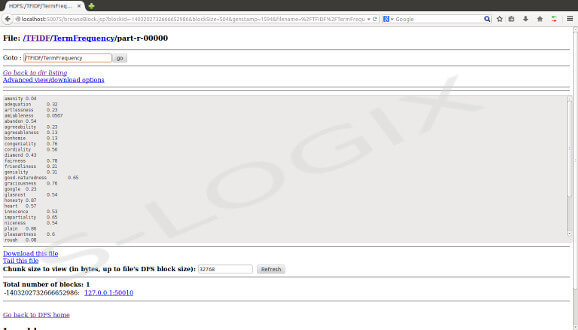• #5, First Floor, 4th Street Dr. Subbarayan Nagar Kodambakkam, Chennai-600 024 Landmark : Samiyar Madam
• pro@slogix.in
• +91- 81240 01111

### How to calculate TF-IDF using MapReduce?

#### Description

According to the term (word) frequency of documents the TF-IDF (Term Frequency- Inverse Term Frequency) estimates the importance of word. In the below code segment, the goal of first Map Reduce job is to count the occurrences of each word in each document. After getting the word frequency, the reducer need to sum the total number of words for each document. In Second MapReduce, the term frequency is calculated and it is passed to third Map Reduce class. The third Map Reduce computes inverse term frequency then it combines both values to calculate score for each word.

###### Sample Code

//First MapReduce

//calculate Term Frequency

//count the number of words in each of the documents in the corpus.

k1-documentId

v1-line of the document

Map Phase input:<k1, v1>

//Extract word from line using StringTokenizer class

k2-word and documetID

v2-1 (word count in MAP should be 1)

output(k2, v2)

Reduce Phase input<k2, List<v2>>

Frequency of word=Sum of the v2 for each key “word and documetID”

k3-word and documetID

v3-Frequency of word

output(k3, v3)

//Second MapReduce

//calculate Term Frequency

//calculate total words for each document

Map Phase input:<k3, v3>

k4-documentID

v4-Frequency of word

output(k4, v4)

Reduce Phase input<k4, List<v4>>

Totalwords for all documents (N)=Sum the total words for all documents (v4)

TermFrequency of word =Frequency of word / N

k5-Word

v5-TermFrequency

output(k5, v5)

//Third MapReduce

//calculate TFIDF

Map Phase input:<k5, v5>

output(k5, v5)

K5-word

v5-TermFrequency of word

Reduce Phase input<k5, v5>

N=Total number of documents in corpus

n=number of documents in corpus where the word appears.

TFIDF=v5 * log(N/n)

k6-Word

v6-TFIDF

output(k6, v6)

###### Screenshots

Input

Document1 file

TermFrequency

TFIDF